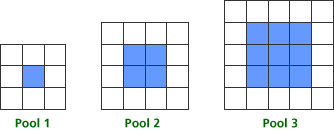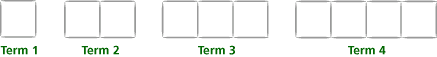# Classroom Case Studies, Grades 6-8 Part B: An Example for Developing Algebraic Thinking (25 minutes)

## Session 10: 6-8, Part B

The National Council of Teachers of Mathematics’ Principles and Standards for School Mathematics (2000) identifies algebra as a strand for grades Pre-K-12. The Standards identify the following concepts that all students should cover and comprehend:
Note 4

 • Understand patterns, relationships, and functions • Represent and analyze mathematical situations and structures using algebraic symbols • Use mathematical models to represent and understand quantitative relationships • Analyze change in various contexts

For the classroom in grades 6-8, understanding patterns includes the following expectations:

 • Represent, analyze, and generalize a variety of patterns with tables, graphs, words, and, when possible, symbolic rules • Relate and compare different forms of representation for relationships • Model and solve contextualized problems using various representations, such as graphs, tables, and equations

In this part, we’ll look at problems that foster algebraic thinking as it relates to these standards, and explore ways of asking questions that elicit algebraic thinking. The situations we’ll be exploring are representative of the kinds of problems you would find in some existing texts; in fact, you may recognize some of them! The goal is for you to examine these problems with the critical eye of someone who has taken this course and is beginning to view algebraic thinking with a different perspective.

Consider the situation below, appropriate for exploration in a grade 6-8 classroom:

Tat Ming is designing square swimming pools. Each pool has a square center that is the area of the water. Tat Ming uses blue tiles to represent the water. Around each pool there is a border of white tiles. Here are pictures of the three smallest square pools that he can design, with blue tiles for the interior and white tiles for the border. Note 4### Problems

Problem B1

Problem B2

How do your questions reflect the algebra content in the situation?

Now focus on the questions you want the students in your classroom to consider. You may want to consider new ways to represent the relationships between the number of tiles of each color and the number of the square pools, and then use those representations to predict what will happen when the pools are very large.

Problem B3

What patterns, conjectures, and questions will your students find as they work with this situation?

Problem B4

What questions could you as the teacher pose to elicit and extend student thinking at your grade level?

Problem B5

Recall the framework you explored in Session 2 in looking at patterns: finding, describing, explaining, and using patterns to predict. Which of these skills will your students use in approaching this problem?

Problem B6

Read the article “Experiences with Patterning” from Teaching Children Mathematics. What ideas mentioned seem appropriate for your classroom? Note 5

Problem B7

In this sequence there are 4 toothpicks in Term 1, 7 toothpicks in Term 2, and 10 toothpicks in Term 3.How many toothpicks are in Term 4? If you continued the pattern, how many toothpicks would you need to make Term 5? Term 6? Term 10?

What questions could you ask to develop students’ skills in describing this pattern?

Problem B8

What questions could you ask to develop students’ skills in predicting?

### Notes

Note 4

Look at NCTM’s recommendations for content in the algebra strand in the Standards.Then look at the problem for designing square swimming pools. After reading the problem, you should work on Problems B1-B5.

Note 5

Read the commentary on the swimming pool problem in “Experiences with Patterning,” by Joan Ferrini-Mundy, Glenda Lappan, and Elizabeth Phillips, in Teaching Children Mathematics (February 1997), p. 282-288.

Now look at the problem on patterns from IMPACT Mathematics, Course 1. Think about asking questions that get at students’ ability to think recursively. For example, if you knew there were 301 toothpicks for Term 100, could you state how many toothpicks were in the 102nd term?

### Solutions

Problem B1

Answers will vary. One question might ask how to use the pictures to help find the relationship between the pool number and the numbers of white and blue tiles.

Problem B2

Answers will vary. For the question above, you might discuss building each pool. For example, the blue part of Pool 1 is a 1-by-1 square and takes one blue tile, the blue part of Pool 2 is a 2-by-2 square and takes four blue tiles, etc.

Problem B3

Answers will vary. At this level, students should be able to write rules for the number of tiles of each color for each pool. The blue tiles are always in the shape of a square, with n2 blue tiles needed for Pool n. The number of white tiles is always a multiple of four, with 4(n + 1) white tiles needed for Pool n.

Problem B4

Answers will vary. At this level, teachers should encourage students to describe the shape of the blue tiles and write a rule to represent the relationship between the pool number and the number of blue tiles. Then ask the students to think about putting white tiles around that blue pool. How many white tiles do you need for the corners? How many more for the bottom and top? Now think about the putting tiles around the sides. How many white tiles does this require in all?

Problem B5

Answers will vary. At this level, students should actually build the pools with two different color square tiles and then describe what they built, thinking of the relationship between the pool number and the number of each color tile needed to build it. The process of building will help students put the patterns into words and symbols. Many students will use all these skills when solving this problem.

Problem B6

Answers will vary. All of the ideas described in the grades 3-4 section are appropriate for this level student. Many students at this level will be able to answer questions posed in the grades 5-6 section.

Problem B7

Answers will vary. Ask students to tell you how to build the 1st term. How many toothpicks will you need? Now describe how to build the 2nd term from the 1st. How many more toothpicks are needed? How many more toothpicks are needed to get from the 10th to the 11th term? Does this same rule work for getting from the 1st to the 2nd term?

Problem B8

Answers will vary. Often students see a rule to get from one entry to the next. Teachers should help students think of ways to connect each entry to its place in the list. Questions like those shown above will help get the rule N = 3n + 1, where N is the number of toothpicks needed to build term n.